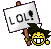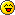# Difference between g and G##Re: Difference between g and G

hey there's an important and amazing difference!!!!universal constant is a constant, which is independent of time, place, velocity, acceleration, or any other parameters. A universal constant has only one value in a single unit system. The value may be different in different unit systems but conversion of each value must yield the same answer. The value of the universal gravitational constant in SI units is 6.674 x 10-11 and the units are Newton meter squared per kilogram squared. The dimensions of the universal gravitational constant can be written as [L]3[T]-2[##Re: Difference between g and G

Hey Guys!! Its interesting!!g vs G

G is the symbol used to identify the universal gravitational constant, g is the symbol used to denote the gravitational acceleration. These two concepts are highly important in the field of gravity. In the study of gravitational fields, these two concepts and symbols are widely applied. It is vital to have a proper understanding in the gravitational acceleration and the universal gravitational constant in order to excel in fields such as physics, classical mechanics, cosmology, astrophysics and even space exploration. In this article, we are going to discuss what gravitational acceleration and universal gravitational constant are, their definitions, values, and dimensions, their applications, the similarities between universal gravitational constant and the gravitational acceleration, and finally the difference between universal gravitational constant and the gravitational acceleration.

g (Gravitational Acceleration)

Gravity is more of a common name used for the concept of the gravitational field. Gravitational field is a concept of a vector field. The gravitational field is in the radial outward direction from the mass. It is measured as GM/r2. G is the universal gravitational constant having the value of 6.674 x 10-11 Newton meter squared per kilogram squared. This gravitational field intensity is also known as gravitational acceleration. The gravitational acceleration is the acceleration of any mass due to the gravitational field. The term gravitational potential is also a part of the definition for the gravitational field. The gravitational potential is defined as the amount of work required to bring a test mass of one kilogram from infinity to the given point. The gravitational potential is always negative or zero, since only gravitational attractions exist, and the work has to be done on an object to bring it closer to the mass, and which is always negative. Gravitational field intensity varies in an inverse square relationship with the distance from the mass.

G (Universal Gravitational Constant)

A universal constant is a constant, which is independent of time, place, velocity, acceleration, or any other parameters. A universal constant has only one value in a single unit system. The value may be different in different unit systems but conversion of each value must yield the same answer. The value of the universal gravitational constant in SI units is 6.674 x 10-11 and the units are Newton meter squared per kilogram squared. The dimensions of the universal gravitational constant can be written as [L]3[T]-2[M]. Quantities such as the mutual gravitational attraction, gravitational acceleration, gravitational field intensity and all other gravitation related quantities are dependent on the universal gravitational constant.

##Difference between g and G

SHARE.................

##Re: Difference between g and G